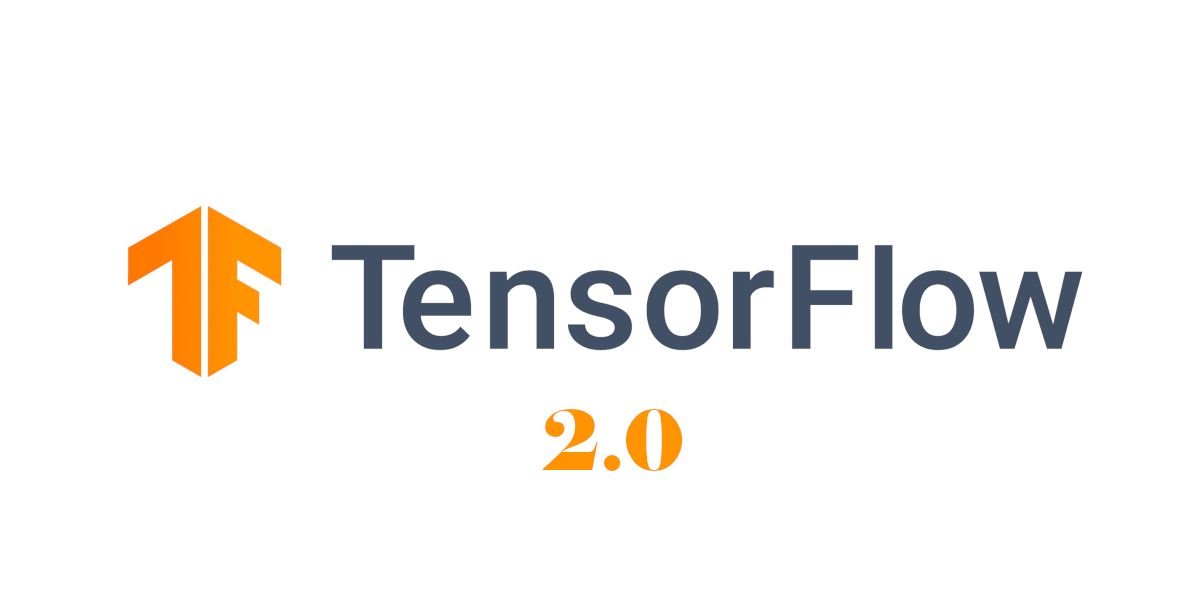# How to Install TensorFlow 2 on Windows 10In this post, I will show you how to install TensorFlow 2 on Windows 10. TensorFlow2 is a free software library used for machine learning applications. It comes integrated with Keras, a neural-network library written in Python. If you want to work with neural networks and deep learning, TensorFlow 2 should be your software of choice because of its popularity both in academia and in industry. Let’s get started!

# Directions

## Install TensorFlow 2

Here are the official instructions for downloading TensorFlow 2, but I will walk you through the process step-by-step.

Open an Anaconda command prompt terminal.

Type the command below to create a virtual environment named tf_2 with the latest version of Python installed. A virtual environment is like an independent Python workspace which has its own set of libraries and Python version installed. For example, you might have a project that needs to run using an older version of Python, like Python 2.7. You might have another project that requires Python 3.7. You can create separate virtual environments for these projects.

`conda create -n tf_2 python`

Press y and then ENTER.

Once the download is finished, activate the virtual environment using this command:

`conda activate tf_2`

Check which version of Python you have installed on your system. I have Python 3.8.0.

`python --version`

Choose a TensorFlow package. I’ll install TensorFlow CPU. Let’s type the following command:

`pip install --upgrade tensorflow`

You might see this error:

ERROR: Could not find a version that satisfies the requirement tensorflow (from versions: none)

ERROR: No matching distribution found for tensorflow

`conda install python=3.6`

Press y and then ENTER.

Check which version of Python you have installed on your system. I have Python 3.6.9 now.

`python --version`

Now install TensorFlow 2.

`pip install --upgrade tensorflow`

Wait for Tensorflow CPU to finish installing. Once it is finished installing, verify the installation by typing:

`python -c "import tensorflow as tf; x = [[2.]]; print('tensorflow version', tf.__version__); print('hello, {}'.format(tf.matmul(x, x)))"`

Here is the output:

You should see your TensorFlow version in the output.

You might see this message:

“I tensorflow/core/platform/cpu_feature_guard.cc:142] Your CPU supports instructions that this TensorFlow binary was not compiled to use: AVX2”

Don’t worry, TensorFlow is working just fine. To get rid of that message, you can set the environment variables inside the virtual environment. Type the following command:

`set TF_CPP_MIN_LOG_LEVEL=2`

Now run this command:

`python -c "import tensorflow as tf; x = [[2.]]; print('tensorflow version', tf.__version__); print('hello, {}'.format(tf.matmul(x, x)))"`

Voila! Message gone.

## Create a Basic Neural Network Using TensorFlow 2

To really see what TensorFlow 2 can do, let’s do the following:

• Build a neural network that classifies images of clothing.
• Train this neural network.
• And, finally, evaluate the accuracy of the model.

We are going to roughly follow the TensorFlow beginner tutorial.

First, install the Matplotlib library.

`pip install matplotlib`

I’m now going to open up a text editor and type a Python program. I will save it to my D drive as fashion_mnist.py. Here is the code:

```from __future__ import absolute_import, division, print_function, unicode_literals

# Import the key libraries
import matplotlib.pyplot as plt
import tensorflow as tf
import numpy as np

# Rename tf.keras.layers
layers = tf.keras.layers

# Print the TensorFlow version
print(tf.__version__)

# Load and prepare the MNIST dataset.
# Convert the samples from integers to floating-point numbers:
mnist = tf.keras.datasets.fashion_mnist
(x_train, y_train), (x_test, y_test) = mnist.load_data()
x_train, x_test = x_train / 255.0, x_test / 255.0

# Let's plot the data so we can see it
class_names = ['T-shirt/top', 'Trouser', 'Pullover', 'Dress', 'Coat', 'Sandal',
'Shirt', 'Sneaker', 'Bag', 'Ankle boot']
plt.figure(figsize=(10,10))

for i in range(25):
plt.subplot(5,5,i+1)
plt.xticks([])
plt.yticks([])
plt.grid(False)
plt.imshow(x_train[i], cmap=plt.cm.binary)
plt.xlabel(class_names[y_train[i]])
plt.show()
```

Within your virtual environment in the Anaconda terminal, navigate to where you saved your code. I will type.

`D:`

Then:

`cd D:\<YOUR_PATH>\install_tensorflow2`

Type dir to see if the Python (.py) file is in that directory.

Now run the code:

`python fashion_mnist.py`

You should see this graphic pop up.

In the terminal window, press CTRL+C on your keyboard to stop the code from running.

Let’s add to our code. Open up the Python file again in the text editor and type the following code. If you are new to neural networks, don’t worry what everything means at this stage.

```from __future__ import absolute_import, division, print_function, unicode_literals

# Import the key libraries
import matplotlib.pyplot as plt
import tensorflow as tf
import numpy as np

# Rename tf.keras.layers
layers = tf.keras.layers

# Print the TensorFlow version
print(tf.__version__)

# Load and prepare the MNIST dataset.
# Convert the samples from integers to floating-point numbers:
mnist = tf.keras.datasets.fashion_mnist
(x_train, y_train), (x_test, y_test) = mnist.load_data()
x_train, x_test = x_train / 255.0, x_test / 255.0

# Let's plot the data so we can see it
class_names = ['T-shirt/top', 'Trouser', 'Pullover', 'Dress', 'Coat', 'Sandal',
'Shirt', 'Sneaker', 'Bag', 'Ankle boot']
plt.figure(figsize=(10,10))

for i in range(25):
plt.subplot(5,5,i+1)
plt.xticks([])
plt.yticks([])
plt.grid(False)
plt.imshow(x_train[i], cmap=plt.cm.binary)
plt.xlabel(class_names[y_train[i]])
plt.show()

# Build the neural network layer-by-layer
model = tf.keras.Sequential()
model.add(layers.Flatten()) # Make the input layer one-dimensional
model.add(layers.Dense(64, activation='relu')) # Layer has 64 nodes; Uses ReLU
model.add(layers.Dense(64, activation='relu')) # Layer has 64 nodes; Uses ReLU
model.add(layers.Dense(10, activation='softmax')) # Layer has 64 nodes; Uses Softmax

# Choose an optimizer and loss function for training:
loss='sparse_categorical_crossentropy',
metrics=['accuracy'])

# Train and evaluate the model's accuracy
model.fit(x_train, y_train, epochs=5)
model.evaluate(x_test,  y_test, verbose=2)
```

Run the code:

`python fashion_mnist.py`

When you see the plot of the clothes appear, just close that window so that the neural network build and run.

Here is the output.

The accuracy of classifying the clothing items was 87.5%. Pretty cool huh! Congratulations! You’ve built and run your first neural network on TensorFlow 2.

To deactivate the virtual environment, type:

`conda deactivate`

Then to exit the terminal, type:

`exit`

At this stage, I encourage you to go through the TensorFlow tutorials to get more practice using this really powerful tool.